## Example Questions

### Example Question #1 : Describing Roles Of Variables & Constants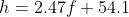Archaeologists use the equation above to estimate the height of fossil hominids from the length of their femurs. If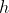represents the estimated height, in centimeters, and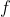represents the measured femur length, in centimeters, what is role of the humber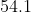in the equation?

The number of centimeters that estimated height increases for each centimeter of length of the femur.

The minimum estimated height of a hominid, regardless of femur size, using this method of estimation.

The average estimated height of a hominid based in this scientific study.

The number of centimeters of femur length that it takes for the overall estimated height to increase one centimeter.

The minimum estimated height of a hominid, regardless of femur size, using this method of estimation.

Explanation:

Note that in this equation, the number 54.1 is a constant: it isn't multiplied by anything. So 54.1 is a minimum number: a femur cannot have a minimum length, so even ifwere to equal zero, the estimated height would be 54.1. This tells you that 54.1 has to be the absolute minimum estimated height of a hominid using this method.

### Example Question #2 : Describing Roles Of Variables & Constants

In preparation for wrestling tryouts, Trey sets a goal of doing 270 pushups per week. The number of pushups he has remaining for the week can be expressed at the end of each day by the equation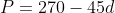, where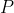is the number of pushups and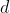is the number of days he has completed his pushups that week. What is the meaning of the value 45 in this equation?

The number of pushups that he does each day.

The number of pushups that he has completed thus far that week.

The number of pushups he has remaining for the week.

The number of days that he has done pushups that week.

The number of pushups that he does each day.

Explanation:

Remember: in word problems, units are always important! In this problem, thinking about units is a great place to start. Since you know that P is a number of pushups, then the other side of the equation needs to be a number of pushups, too, so that the units match. And three of the four items in the equation are given to you:

P = Number of pushups left
270 = Total number of pushups
d = Days that Trey has done pushups

That alone helps you use process of elimination, since "number of days he's already done pushups" and "number of pushups remaining" are already defined by other parts of the equation. And it should also help you think your way through it. Without using the test’s variables, you could set up your own equation:

Pushups left = Total - Pushups he’s already done

And since “pushups he’s already done” is represented mathematically as 45d, you can eliminate that answer since 45 itself doesn’t equal that overall value – it needs to be multiplied by d. Or, as before, you can look at the 45d portion as a recurring amount. d = the number of days, and if you multiply the number of days by the number of pushups per day, you’ll get the total amount of pushups over that period. So 45 is the number of pushups that he does each day.

### Example Question #3 : Describing Roles Of Variables & Constants

A taxi service calculates its fares using the formula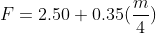, where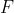is the total fare and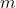is the total number of miles of the trip. Which of the following best describes the role of the number 2.50?

The number of quarter-miles driven on the trip

The number of miles driven on the trip

The up-front fee charged regardless of miles driven

The charge for each mile driven on the trip

The up-front fee charged regardless of miles driven

Explanation:

Notice that the number 2.50 isn’t multiplied by anything, so it’s not a recurring charge of any kind. Even if m = 0, that 2.50 remains unchanged, so 2.50 is the charge that happens regardless of the distance. This tells you that 2.50 is the up-front fee that is charged regardless of the number of miles on the trip.

### Example Question #3 : Describing Roles Of Variables & Constants

A beekeeper models the number of bees in his colony by the equation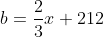, where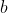represents the number of bees in the colony and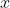represents the number of months since he began his colony. Based on the model, which of the following statements are true?

I. The number of bees in the colony is decreasing with time.

II. The colony began with over 200 bees.

III. The colony gains an average of 8 bees per year.

II and III only

I, II, and III

I and III only

I and II only

II and III only

Explanation:

On occasion, the SAT will present you with problems like this one, in which you have to determine what must be true for up to three different statements. These tend to be a bit more time consuming, because they’re essentially three problems in one.

Looking at the given equation,, analyze the role of each term.is the total number of bees at any given point of time.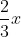says that for every month, the number of bees increases by approximately 2/3 (note that this is the beekeeper's model, not an exact number, so it's okay that the number of bees isn't always an integer)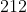is a constant, and ifwere 0 the total number of bees would be 212. So this must mean that there were 212 bees to start.

If you then look at the statements:

I is not true, as the number of bees is increasing with each month.

II is true; the beginning number was 212.

III is true; if the number of bees grows by 2/3 each month, and there are 12 months in a year, then the number of bees is growing by an estimated 8 bees each year.

### Example Question #4 : Describing Roles Of Variables & Constants

Hannah is selling candles for a school fundraiser all fall. She sets a goal of selling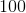candles per month. The number of candles she has remaining for the month can be expressed at the end of each week by the equations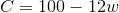, where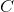is the number of candles and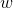is the number of weeks she has sold candles this month. What is the meaning of the value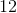in this equation?

The number of candles that she has sold thus far that week.

The number of weeks that she has sold candles this month.

The number of candles she has remaining for the month.

The number of candles that she sells each week.

The number of candles that she sells each week.

Explanation:

Since we know thatstands for weeks, the answer has to have something to do with the weeks. This eliminates "the number of candles she has remaining for the month." Also, we can eliminate "the number of weeks that she has sold candles this month" because that would be our value for, not what we'd multiplyby. The correct answer is,  "the number of candles that she sells each week."

### Example Question #6 : Describing Roles Of Variables & Constants

Upon discovering a tree virus in British Columbia, scientists created a model to predict the tree population in a certain forest: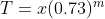, where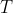represents he number of trees aftermonths since the virus's discovery, andrepresents the original number of trees that existed in the forest when the scientists made the discovery. Assuming that the model is correct, which of the following best describes the impact of the virus on the forest?

The number of trees in the forest is decreasing by 73 each month.

The number of trees in the forest is decreasing by 27 each month.

The number of trees in the forest is decreasing by 27% each month.

The number of trees in the forest is decreasing by 73% each month.

The number of trees in the forest is decreasing by 27% each month.

Explanation:

As you can see from the answer choices to the first question, you need to make two important determinations:

• Is this an exponential decrease, or a linear decrease?

• Should the number in question be 73 or 27?

When you’re determining whether a change is exponential or linear, look to see if there is an exponent. The exponent,, just outside the parentheses tells you that this is an exponential function. What does that mean? That the loss of 27% per month compounds each month. Exponential increases and decreases mean that the increase or decrease is taken as a percent of the new value each time; linear increases and decreases mean that the same amount is increased or decreased each month, because that percent change is taken from the original value. So this is an exponential change, meaning that the answer must be one of the percentages, not one of the numbers.

The fact that this is a decrease tells you what is happening inside the parentheses. For an exponential decrease, the formula is:

New value = (Original Value)(1 - rate)(number of periods)

The rate is a percentage expressed as a decimal, and in a decrease problem that rate is always subtracted from 1. This is because you’re calculating the number of items (in this case trees) remaining, so you need to convert the decrease into a function of the number that you keep, not the number that you’re losing. Think of it like a discount at a store: if an item is 20% off, you pay the other 80% of the price that’s remaining. So here, the expression shows that 73% of the trees remain each month, meaning that the forest is losing 27% of its trees each month (1−0.27=0.73). Therefore, the correct answer is "the number of trees is decreasing by 27% each month."

### Example Question #5 : Describing Roles Of Variables & Constants

Shawn is purchasing t-shirts for himself and the members of his soccer team. The online vendor uses the formula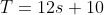to represent the total cost a customer pays, inclusive of the price of each shirt plus a one-time shipping fee that is the same regardless of the number of shirts purchased. Ifrepresents the total amount that Shawn will pay for purchasing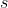shirts, what is the role of the number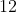in this formula?

The total cost that Shawn pays before the shipping cost is applied

The total number of shirts he purchases

The price he pays for each shirt

The amount of the shipping fee

The price he pays for each shirt

Explanation:

When you see formulas like this that express the total cost for a number of items, there are some standard components you should recognize. Whenever there is a plain number added, with no variable next to it (as you see with + 10 here), that is a fixed charge that does not depend on the number of items purchased.  Where you do see a number multiplied by a variable, like with 12s here, that represents the amount of money that depends on the number of items purchased: the numerical coefficient is the cost-per-item, and the variable is the number of items. Which should make logical sense - the shopper gets to choose the number of items to buy, so that number can vary customer to customer, making a "variable" appropriate there. Here you're asked about the number 12, which is multiplied by the variable s - that means that 12 is the price per shirt, with s being the number of shirts Shawn purchases.

### Example Question #6 : Describing Roles Of Variables & Constants

A pizzeria uses the equation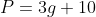to predict the number of pizzas,, that will be ordered for delivery in any given hour based on the number of football games,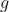, that are being televised during that hour. What is the meaning of the value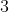in this equation?

The number of pizzas that would be ordered if no football games were being televised

The number of additional pizzas ordered for delivery per football game being televised

The number of football games being televised in a typical hour

The number of pizzas ordered for delivery each hour

The number of additional pizzas ordered for delivery per football game being televised

Explanation:

In this equation you know thatis the total number of pizzas and thatis the number of football games. Think about what happens: ifincreases, there are more pizzas ordered.tells us how many extra pizzas are ordered for each game that is being televised. The pizzeria would sell 10 pizzas if no games were on TV, 13 if one is on, 16 if two is on, etc.  Sois the number of additional pizzas expected to be ordered per football game on TV.

### Example Question #7 : Describing Roles Of Variables & Constants

Researchers have modeled the extent of ice loss in Greenland using the equation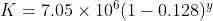, where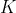represents the surface area of Greenland covered by ice, in square kilometers, measured on September 30 of each year,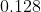represents the 12.8% rate at which ice is lost each year, and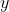represents the number of years that have elapsed since the study began in 1979. What is the meaning of the number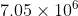in this equation?

The number of square kilometers of ice in Greenland as of September 30, 1979

The average percent decrease in ice in Greenland each year since 1979

The number of square kilometers of ice remaining in Greenland

The number of square kilometers of ice that Greenland loses each year

The number of square kilometers of ice in Greenland as of September 30, 1979

Explanation:

An exponential change calculation generally has three components: the initial amount, the percent change, and the number of periods that have undergone that change. The typical structure of such a calculation is:

New Amount = Original Amount(1 +/- Percent Change As A Decimal)Number of Periods

Here we're dealing with a 12.8% decrease, so within the parentheses the percentage is subtracted, andhas been identified as the number of periods.is the original amount that gets multiplied by the percent change taken to its exponent, so the correct answer is the original amount, which in this problem is defined as "The number of square kilometers of ice in Greenland as of September 30, 1979."

### Example Question #8 : Describing Roles Of Variables & Constants

Sarah has created an equation to model the amount of money in her savings account. Her net income is $50,000 per year, and her expenses each month are$2,000, so she models her savings at the end of each month using the equation: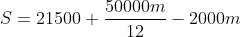In this equation,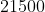represents the \$21,500 she had in savings when she first began tracking her savings this way, andrepresents the number of months that it has been since she started. What is the meaning of the numberin this calculation?

12 is the number of months that she has been working at her current job

Her goal is to earn 12 times as much money as she spends

After 12 months she expects to hit her savings goal

She divides by 12 to convert her annual salary to a monthly amount

In this problem, Sarah's income is quoted as an annual amount but her expenses are quoted as a monthly amount. In order to calculate her savings, she needs those units to both be in either "per year" or "per month." Since the variablealso represents a number of months, it is necessary for her to convert her annual salary into a monthly amount so that she can properly subtract expenses from income to reflect the amount of money saved. Therefore, dividing by 12 is how she makes that conversion.